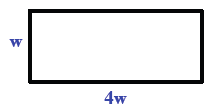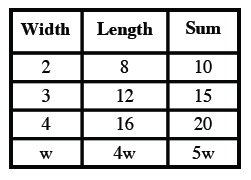Home > INT3 > Chapter 1 > Lesson 1.2.2 > Problem1-85

1-85.

Write one or two equations to solve the following problem.

A rectangle's length is four times its width. The sum of its two adjacent sides is $22$ cm. How long is each side? Homework Help ✎

Draw and label a picture or make a table of values to get started.$(4w) + w = 22$
$5w = 22$